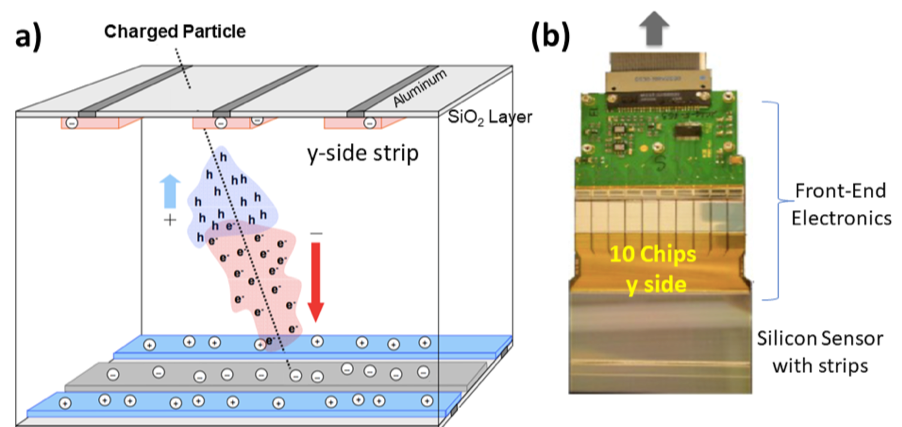# The Silicon Tracker

The tracker, together with the magnet, measures the momentum $p$, charge $Z$, and rigidity $R=p/Z$, of charged cosmic rays.  The tracker has nine layers, see Figure 1, the first (L1) at the top of the detector, the second (L2) just above the magnet, six (L3 to L8) within the bore of the magnet, and the last (L9) just above the ECAL.  L2 to L8 constitute the inner tracker.  Each layer contains double-sided silicon microstrip detectors that independently measure the x and y coordinates as shown schematically in Figure 2 along with the front-end electronics.  The tracker accurately determines the particle trajectory by multiple measurements of the coordinates with a resolution in each layer of better than 10 μm in the bending direction. The inner tracker is held stable by a carbon fiber structure with negligible coefficient of thermal expansion. The stability of the inner tracker is monitored using 20 IR laser beams that penetrate layers L2 through L8 and provide submicron position measurements. Using cosmic rays over a 2 minute window, the position of L1 is aligned with a precision of 5 μm with respect to the inner tracker and L9 with a precision of 6 μm.  The maximum detectable rigidity, MDR, for $|Z|=1$ particles is 2 TV over the 3 m lever arm from L1 to L9.  Each layer of the tracker also provides an independent measurement of Z. The charge resolution of the layers of the inner tracker together is $\Delta Z=0.05$ for $Z=1$.Figure 1.  Schematic of the AMS tracker and its alignment system with 20 IR lasers for the inner tracker, layers L2 to L8.  The outer tracker layers, L1 and L9, are aligned every two minutes with cosmic rays.  The alignment accuracy is 5 microns for L1 and 6 microns for L9.Figure 2. (a) Schematic of the measurement principles of the silicon tracker.  (b) The front-end electronics attached to the strips.

Before launch, AMS was extensively calibrated at the CERN SPS with 180 and 400 GeV/c proton beams and positron, electron, and pion beams of 10 to 290 GeV/c.  In total, calibrations with 18 different energies and particles at 2000 positions were performed.  These data allow the determination of the tracker rigidity resolution function with high precision and the verification of the absolute rigidity scale as shown in Figure 3. The vast majority of the tracks are measured within the core of the resolution with tails due to scattering.  The figure also shows that the performance is precisely reproduced by the Monte Carlo simulation, both in the core and in the tails.Figure 3. Comparison of the tracker resolution for $|Z|=1$ measured with 400 GeV/c protons in the test beam and the Monte Carlo simulation.

Figure 4 shows the alignment stability of (a) Layer 1 and (b) Layer 9 over seven years.  As seen, the alignment stability is 2.2 microns for Layer 1 and 2.3 microns for Layer 9.  Note, as seen, in October and November 2014, the tracker was partially switched off to study the overall thermal system.Figure 4. The alignment stability of (a) Layer 1 and (b) Layer 9 over seven years.